• 最近微信上的那个给红包让看图片的小功能真的是异常火爆啊，我也忍不住去弄了一波，昨晚...从整个过程来看，无疑最主要的就是这个图像模糊操作了，具体可以使图像模糊的方法很多，这里主要讲讲原理：我们都知道，彩色
最近微信上的那个给红包让看图片的小功能真的是异常火爆啊，我也忍不住去弄了一波，昨晚在看博客的时候发现有大牛发了一个相关的原理解释，我看完之后，赶紧自己记录学习一下。

让我们来回顾下微信上的这个小功能的操作：在没点击前，图像是一种模糊状态，然后点击给红包之后，那么就可以看到真正的图片了。从整个过程来看，无疑最主要的就是这个图像的模糊操作了，具体可以使图像模糊的方法很多，这里主要讲讲原理：

我们都知道，彩色图像是一个三通道的RGB图像，R，G，B分别有0-255这么一个取值区间，现在我们仅仅就一个通道进行说明，可以进行类推。
我们来看看图像模糊是什么一个概念，首先，图像中的像素每一个都有自己的像素值，这些像素值决定了图像最终的显示。来想象一幅模糊的图，是不是感觉越模糊，这些像素点之间的差别就越小呢？确实是这样，这也是图像模糊的原理，也就是一种像素的平滑化，通过对图像中的像素值进行平均处理，让这些像素值越来越来接近，来达到一种人尽量无法辨识出这些像素点的差别，从来产生模糊的效果。

原理就是如此，从原理上，我们还可以得到一个启示，那就是对于取均值的半径大小越大，那么整个模糊效果也就越好，很好理解，因为取的范围越大，意味着相似的像素点越多，达到的模糊效果也就越好了。

那么具体有什么方法去取均值呢？

最简单的方法就是直接取所需处理像素值的周边半径内平均来作为它自身的像素信息，对图像内所有像素点都可以这么处理，另外边界点需要另外找处理方法。

还可以采取正态分布取权值的做法来取平均值，因为实际上围绕一个特定的像素点，离它越远，和它的相似度就越低，对它们处理的权重也应该越低，而正态分布恰好是一个山型函数可以围绕着这一个特定的像素点取半径进行处理。

然而实际情况下，正态分布虽说有理有据，但是效率不是很高，所以可以取多次周边平均的方法来增加模糊效果。当然针对正态分布本身也可以进行优化，方法很多，我也只是大概了解了一下，有兴趣及需求的朋友可以自行去深入学习。
展开全文图片
• ， 这个应用可以在很大程度上消除相机拍摄图像的抖动模糊。效果图如下： 效果虽然不算完美，但是也很令人惊讶。 一直以来对这个领域了解不是很多，今天看到这个，就在网上搜索

原文地址：http://www.bfcat.com/index.php/2012/10/deblur/

今天看到新浪微博上面转发的一个应用叫做 Enhance！， 这个应用可以在很大程度上消除相机拍摄图像的抖动模糊。效果图如下：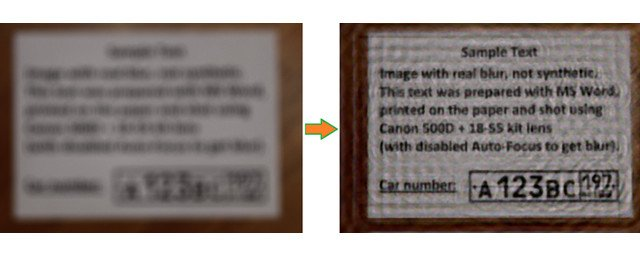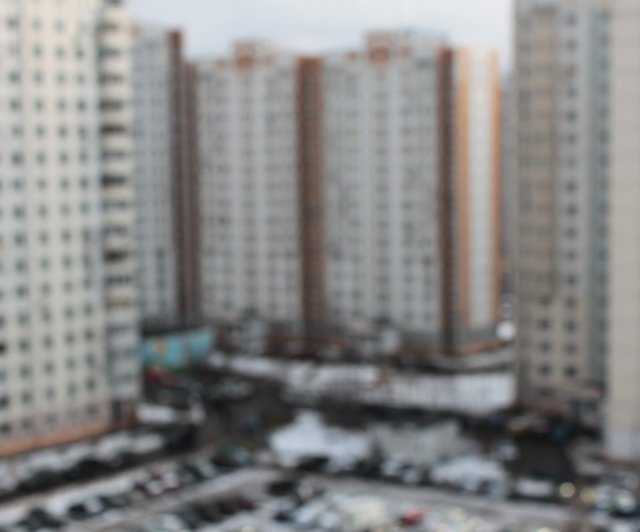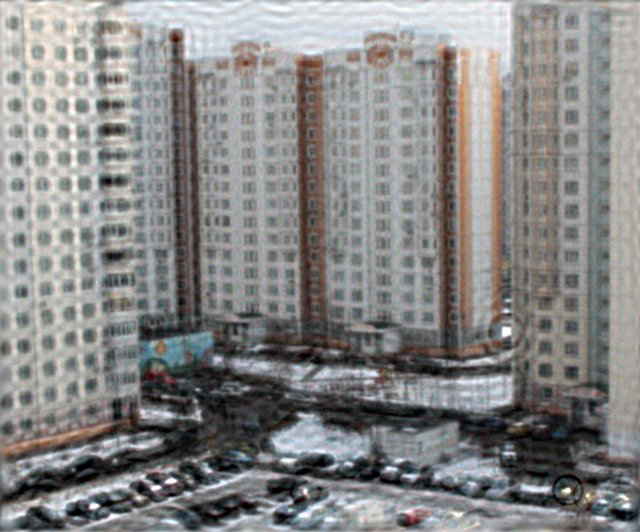效果虽然不算完美，但是也很令人惊讶。

一直以来对这个领域了解不是很多，今天看到这个，就在网上搜索了一下这方面的资料。看到香港中文大学的Jiaya Jia以及美国哥伦比亚大学CAVE实验室这方面的研究主页。

下面两个都是Jiaya Jia的项目主页：

http://www.cse.cuhk.edu.hk/~leojia/projects/motion_deblurring/index.html

http://www.cse.cuhk.edu.hk/~leojia/projects/robust_deblur/index.html

没有仔细看他们的论文，但是通过一些介绍，可以了解到，简单的图像模糊可以看作是模糊核与原始图像进行了卷积，因此模糊核估计是图像去模糊的一个重要部分。如果模糊核已知，那么算法就退化为Non-blind Image Deconvolution。

Jiaya Jia的方法主要是针对一张已经有了运动模糊的图像，算法分为 模糊核估计以及图像恢复两个步骤，这两步构成了一个迭代优化框架。即首先估计模糊核，然后对图像恢复，然后重新估计模糊核，恢复图像，最终直到收敛。他们的另一个主要贡献在于分析了造成图像模糊的多种因素，进而提出了估计模糊核更好的概率模型。

这是他们在SIGGRAPH2008的文章结果之一，效果已经相当好了。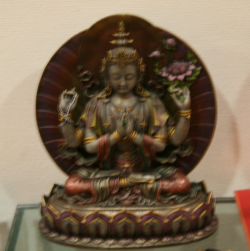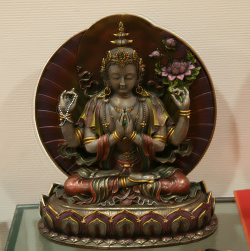哥伦比亚大学的这个项目主页要更老一些，2004年的

http://www1.cs.columbia.edu/CAVE/projects/motion_deblur/motion_deblur.php

这个项目中，作者发明了一种混合相机，通过均衡时间分辨率与空间分辨率来进行相机运动的预测，从而更好的估计出点扩散方程（PSF）。也就是运动模糊的模糊核。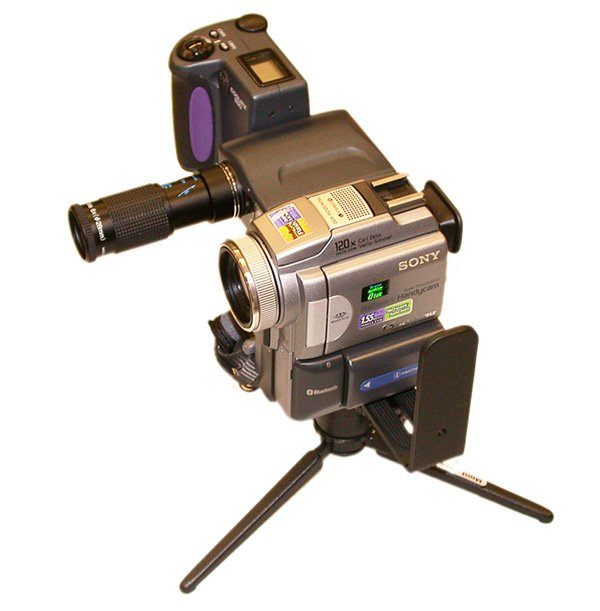展开全文图像处理
• 1.对焦模糊 ... 为了便于用数学语言描述图像及其变换，现作如下规定：图像的左上角为坐标原点(0,0)，图像的长度方向为x轴，宽度方向为y轴，整个图像落在第一象限。  假设无任何模糊和噪声的...
1.对焦模糊

均值滤波

高斯滤波

2.运动模糊

运动模糊的数学原理

运动模糊，是在拍摄设备快门打开的时间内，物体在成像平面上的投影发生平移或旋转，使接受的影像彼此发生重叠。

为了便于用数学语言描述图像及其变换，现作如下规定：图像的左上角为坐标原点(0,0)，图像的长度方向为x轴，宽度方向为y轴，整个图像落在第一象限。

假设无任何模糊和噪声的真实图像为f(x,y)，模糊图像为g(x,y)。由于运动模糊是由图像彼此重叠造成的，所以成立：

其中，Cx为图像在方向上的平移速度，Cy为在方向上的平移速度，T为快门打开时间即产生模糊图像的时间，n(x,y)为加性噪声。

计算机实现细节

为了简化计算过程，我假设只有运动模糊而没有任何加性噪声，而且产生模糊的运动是沿x方向的。

对于真实图像，模糊图像自然是原始图像的叠加，但对于数字图像，由于像素信息由数值表示，不能简单地将相应像素值相加，而是将像素信息缩小后相加，否则会使亮度成倍增加，使图像严重失真。

cv::Mat motionblur(cv::Mat in , unsigned int steps_x, unsigned int steps_y)
{
// steps_x steps_y should >= 2.
int rows = in.rows;
int cols = in.cols;
int channel = in.channels();
cv::Mat out(rows, cols, CV_8UC(channel));
int element = cols * channel;

if (steps_x != 0) {
for (size_t i = 0; i < rows; i++)
{
uchar * ptr_in = in.ptr<uchar>(i);
uchar * ptr_out = out.ptr<uchar>(i);
float sum_b = 0, sum_g = 0, sum_r = 0;
for (size_t j = 0; j < element; j += channel)
{
if ((j / channel) < steps_x) {
if (channel == 3) {
sum_b += ptr_in[j];
sum_g += ptr_in[j + 1];
sum_r += ptr_in[j + 2];

ptr_out[j] = static_cast<uchar>(sum_b / ((j / channel) + 1));
ptr_out[j + 1] = static_cast<uchar>(sum_g / ((j / channel) + 1));
ptr_out[j + 2] = static_cast<uchar>(sum_r / ((j / channel) + 1));
}
else if (channel == 1) {
sum_b += ptr_in[j];
ptr_out[j] = static_cast<uchar>(sum_b / (j + 1));
}

}
else {
if (channel == 3) {
sum_b = sum_b + ptr_in[j] - ptr_in[j - steps_x * channel];
sum_g = sum_g + ptr_in[j + 1] - ptr_in[j - steps_x * channel + 1];
sum_r = sum_r + ptr_in[j + 2] - ptr_in[j - steps_x * channel + 2];

ptr_out[j] = static_cast<uchar>(sum_b / steps_x);
ptr_out[j + 1] = static_cast<uchar>(sum_g / steps_x);
ptr_out[j + 2] = static_cast<uchar>(sum_r / steps_x);
}
else if (channel == 1) {
sum_b = sum_b + ptr_in[j] - ptr_in[j - steps_x];
ptr_out[j] = static_cast<uchar>(sum_b / steps_x);
}

}
}
}
}

if (steps_y != 0) {
for (size_t i = 0; i < cols; i++)
{
float sum_b = 0, sum_g = 0, sum_r = 0;
for (size_t j = 0; j < rows; j++)
{
if (j < steps_y) {
if (channel == 3) {
sum_b += in.at<cv::Vec3b>(j, i);
sum_g += in.at<cv::Vec3b>(j, i);
sum_r += in.at<cv::Vec3b>(j, i);

out.at<cv::Vec3b>(j, i) = static_cast<uchar>(sum_b / (j + 1));
out.at<cv::Vec3b>(j, i) = static_cast<uchar>(sum_g / (j + 1));
out.at<cv::Vec3b>(j, i) = static_cast<uchar>(sum_r / (j + 1));
}
else if(channel == 1){
sum_b += in.at<uchar>(j, i);
out.at<uchar>(j, i) = static_cast<uchar>(sum_b / (j + 1));
}
}
else {
if (channel == 3) {
sum_b = sum_b + in.at<cv::Vec3b>(j, i) - in.at<cv::Vec3b>(j - steps_y, i);
sum_g = sum_g + in.at<cv::Vec3b>(j, i) - in.at<cv::Vec3b>(j - steps_y, i);
sum_r = sum_r + in.at<cv::Vec3b>(j, i) - in.at<cv::Vec3b>(j - steps_y, i);

out.at<cv::Vec3b>(j, i) = static_cast<uchar>(sum_b / steps_y);
out.at<cv::Vec3b>(j, i) = static_cast<uchar>(sum_g / steps_y);
out.at<cv::Vec3b>(j, i) = static_cast<uchar>(sum_r / steps_y);
} else if(channel == 1) {
sum_b = sum_b + in.at<uchar>(j, i) - in.at<uchar>(j-steps_y, i);
out.at<uchar>(j, i) = static_cast<uchar>(sum_b / steps_y);
}
}

}
}
}
return out;
}

实现效果

原图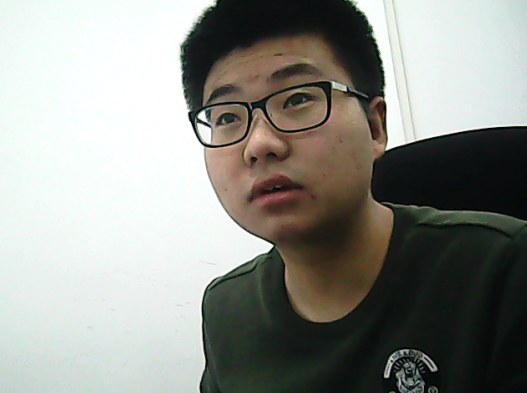处理之后的图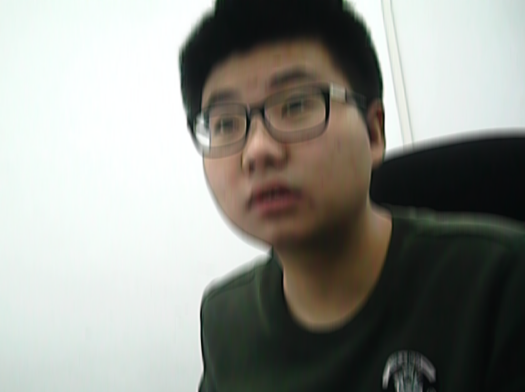3.径向模糊

（与运动模糊原理一致）

PS里称为 径向模糊->缩放， 还有一种 径向模糊->旋转。

缩放的原理:

确定一个中心点(如0.5, 0.5), 跟当前像素连一条线. 以当前像素为中心, 在线上的附近像素进行采样, 最后取一下平均值.

同样，旋转的原理相似，就是相同半径的一定邻域内的均值。

缩放时，半径变化，旋转时，角度变化。

使用OpenCV实现

原图：径向模糊->缩放：

#include <math.h>
#include <opencv/cv.h>
#include <opencv/highgui.h>

using namespace cv;
using namespace std;

int num=40;//num：均值力度;

int main()
{
Mat src1u;
split(src,src1u);

int width=src.cols;
int heigh=src.rows;
Mat img;
src.copyTo(img);
Point center(width/2,heigh/2);

for (int y=0; y<heigh; y++)
{

uchar *imgP  = img.ptr<uchar>(y);

for (int x=0; x<width; x++)
{
int R = norm(Point(x,y)-center);
double angle = atan2((double)(y-center.y),(double)(x-center.x));

int tmp0=0,tmp1=0,tmp2=0;

for (int i=0;i<num;i++)        //num：均值力度 ，i为变化幅度;
{
int tmpR = (R-i)>0?(R-i):0;

int newX = tmpR*cos(angle) + center.x;
int newY = tmpR*sin(angle) + center.y;

if(newX<0)newX=0;
if(newX>width-1)newX=width-1;
if(newY<0)newY=0;
if(newY>heigh-1)newY=heigh-1;

tmp0 += src1u.at<uchar>(newY,newX);
tmp1 += src1u.at<uchar>(newY,newX);
tmp2 += src1u.at<uchar>(newY,newX);

}
imgP[3*x]=(uchar)(tmp0/num);
imgP[3*x+1]=(uchar)(tmp1/num);
imgP[3*x+2]=(uchar)(tmp2/num);
}

}
imshow("径向模糊",img);
waitKey();
imwrite("D:/径向模糊（缩放）.jpg",img);
}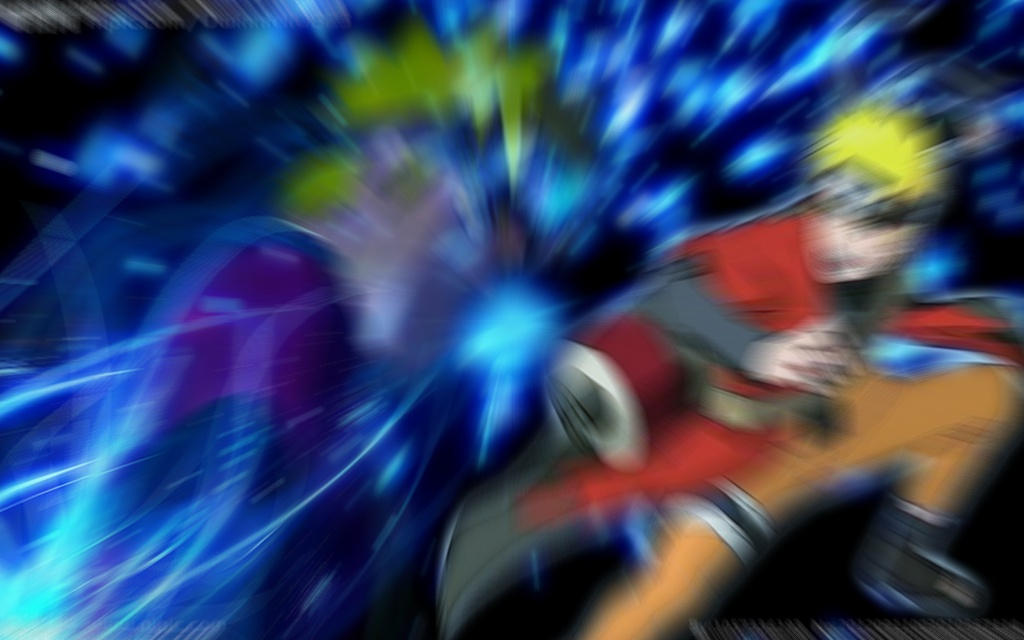径向模糊->旋转：

#include <math.h>
#include <opencv/cv.h>
#include <opencv/highgui.h>

using namespace cv;
using namespace std;

int num=20;    //均值力度;

int main()
{
Mat src1u;
split(src,src1u);

int width=src.cols;
int heigh=src.rows;
Mat img;
src.copyTo(img);
Point center(width/2,heigh/2);

for (int y=0; y<heigh; y++)
{

uchar *imgP  = img.ptr<uchar>(y);

for (int x=0; x<width; x++)
{
int R = norm(Point(x,y)-center);
double angle = atan2((double)(y-center.y),(double)(x-center.x));

int tmp0=0,tmp1=0,tmp2=0;

for (int i=0;i<num;i++)    //均值力度;
{

angle+=0.01;        //0.01控制变化频率，步长

int newX = R*cos(angle) + center.x;
int newY = R*sin(angle) + center.y;

if(newX<0)newX=0;
if(newX>width-1)newX=width-1;
if(newY<0)newY=0;
if(newY>heigh-1)newY=heigh-1;

tmp0 += src1u.at<uchar>(newY,newX);
tmp1 += src1u.at<uchar>(newY,newX);
tmp2 += src1u.at<uchar>(newY,newX);

}
imgP[3*x]=(uchar)(tmp0/num);
imgP[3*x+1]=(uchar)(tmp1/num);
imgP[3*x+2]=(uchar)(tmp2/num);
}

}
imshow("径向模糊",img);
waitKey();
imwrite("D:/径向模糊(旋转).jpg",img);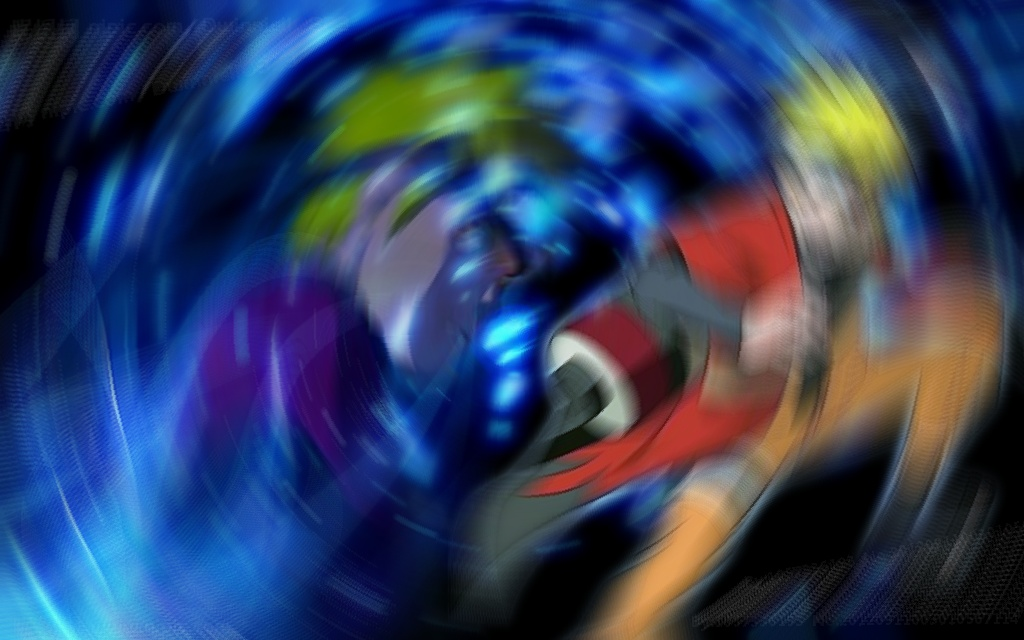展开全文• 高斯模糊是一种两维的卷积模糊操作，在图像完成高斯模糊相对于均值模糊来说， 计算量会增加，但是高斯模糊可以实现一些特殊效果，特别是在图像噪声(非椒盐 噪声)消去方面，更是有着非常好的效果。一维高斯公式如下...
高斯模糊是一种两维的卷积模糊操作，在图像完成高斯模糊相对于均值模糊来说，
计算量会增加，但是高斯模糊可以实现一些特殊效果，特别是在图像噪声(非椒盐
噪声)消去方面，更是有着非常好的效果。一维高斯公式如下：其中x是制定[-n,n]范围的整数，sigma代表标准方差。通常取值为1.
一维高斯函数Java代码如下：
	private float[] get1DKernalData(int n, float sigma) {
float sigma22 = 2*sigma*sigma;
float Pi2 = 2*(float)Math.PI;
float sqrtSigmaPi2 = (float)Math.sqrt(Pi2) * sigma ;
int size = 2*n + 1;
int index = 0;
float[] kernalData = new float[size];
for(int i=-n; i<=n; i++) {
float distance = i*i;
kernalData[index] = (float)Math.exp((-distance)/sigma22)/sqrtSigmaPi2;
System.out.println("\t" + kernalData[index]);
index++;
}
return kernalData;
}
假设输入 n= 1, sigma = 1时，输出的Kernel数据为：
0.24197073, 0.3989423,
0.24197073

两维的高斯分布函数为：对应的Java实现代码为：
	public float[][] get2DKernalData(int n, float sigma) {
int size = 2*n +1;
float sigma22 = 2*sigma*sigma;
float sigma22PI = (float)Math.PI * sigma22;
float[][] kernalData = new float[size][size];
int row = 0;
for(int i=-n; i<=n; i++) {
int column = 0;
for(int j=-n; j<=n; j++) {
float xDistance = i*i;
float yDistance = j*j;
kernalData[row][column] = (float)Math.exp(-(xDistance + yDistance)/sigma22)/sigma22PI;
column++;
}
row++;
}

for(int i=0; i<size; i++) {
for(int j=0; j<size; j++) {
System.out.print("\t" + kernalData[i][j]);
}
System.out.println();
System.out.println("\t ---------------------------");
}
return kernalData;
}
当n=1, sigma=1时对应输出的Kernel数据为：
0.058549833   0.09653235     0.058549833
0.09653235     0.15915494     0.09653235
0.058549833   0.09653235     0.058549833
一个2D高斯分布的图可以表示如下：高斯过滤在图像处理是一种低通滤波，会除去图像的细节而保持整体不变化，在图像美化和特效
方面，高斯过滤有这很多应用。高斯模糊不同于均值模糊！

本文实现完整的高斯模糊算法包括下面几个步骤：
1. 生成高斯操作数即Kernel Data
2. 从图像中读取像素，利用第一步的操作数，完成卷积。
3. 发现图像处理前后的最大像素值peak得出rate
4. 完成归一化操作，返回处理后像素数组

关键程序解析：
利用操作数完成卷积的代码参看以前的Blog文章《图像处理之理解卷积》
完成归一化操作的算法非常简单, 主要是利用第三步计算出来的rate
        // normalization
float rate = inMax/outMax;
System.out.println("Rate = " + rate);
for(int row=0; row<height; row++) {
for(int col=0; col<width; col++) {
index = row * width + col;
int rgb1 = tempoutPixels[index];
int red = (rgb1 >> 16) & 0xff;
int green = (rgb1 >> 8) & 0xff;
int blue = rgb1 & 0xff;
red = (int)(rate * red);
green = (int)(rate * green);
blue = (int)(rate * blue);
outPixels[index] = (rgb1 & 0xff000000) | (red << 16) | (green << 8) | blue;
}
}
高斯模糊效果如下：- 左边为原图                                                                                                                                                            - 右边为高斯模糊之后效果，发现皱纹和手部滑了

等等现在还不最cool的效果，高斯模糊之后如果与原图像叠加会出现一种Glow的
效果，好像灯光打在图像上一样，Glow处理之后的运行效果如下：
原图：实现Glow Filter之后的图像：实现Glow算法只是高斯模糊输出像素值叠加原来的像素值。
		int index = 0;
for ( int y = 0; y < height; y++ ) {
for ( int x = 0; x < width; x++ ) {
int rgb1 = outPixels[index];
int r1 = (rgb1 >> 16) & 0xff;
int g1 = (rgb1 >> 8) & 0xff;
int b1 = rgb1 & 0xff;

int rgb2 = inPixels[index];
int r2 = (rgb2 >> 16) & 0xff;
int g2 = (rgb2 >> 8) & 0xff;
int b2 = rgb2 & 0xff;

r1 = PixelUtils.clamp( (int)(r1 + a * r2) );
g1 = PixelUtils.clamp( (int)(g1 + a * g2) );
b1 = PixelUtils.clamp( (int)(b1 + a * b2) );

inPixels[index] = (rgb1 & 0xff000000) | (r1 << 16) | (g1 << 8) | b1;
index++;
}
}
转载时请注明出处！！，谢谢！
展开全文图像处理 float 算法 java
• 图像的模糊图像处理中比较常用和简单的处理手段之一。那么使用这种手段的原因就是为了给图像进行去噪，便于后面的处理。 模糊处理的背后的原理是什么呢，其实就是卷积计算。而在高等数学里面，卷积是连续的，在...
图像的模糊是图像处理中比较常用和简单的处理手段之一。那么使用这种手段的原因就是为了给图像进行去噪，便于后面的处理。

模糊处理的背后的原理是什么呢，其实就是卷积计算。而在高等数学里面，卷积是连续的，在图像处理中，我们所说的卷积是离散的。直接来上图你就清楚了卷积是怎么回事。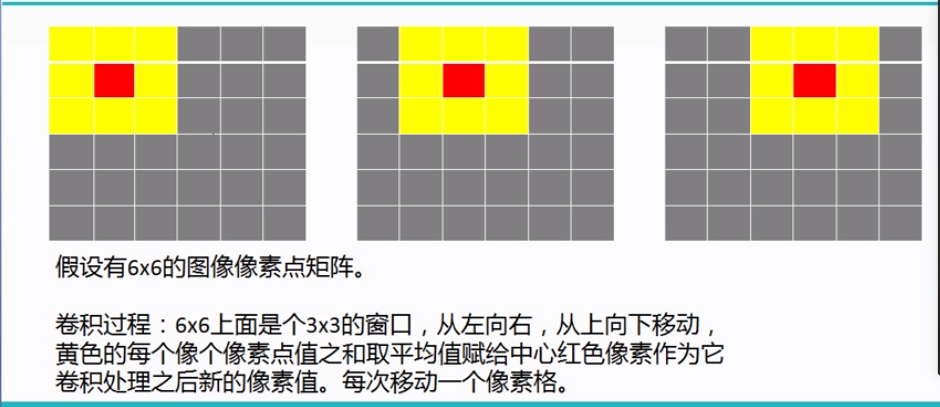你看是不是我们之前讲的图像的掩模很相似。简单的说，拿一个小方格在图像这个方格上进行滑动，其实这个和深度学习里面的卷积是差不多的原理。这样我们就可以得到一个新的图像了。

所以你看，不同类型的模糊处理背后其实是对应不同的小方格，也就是俗称的滤波器或者卷积核。

根据原理的不同，模糊方式有很多种，我们来介绍比较常用的几种。

1、均值滤波。(blur)

均值滤波从字面意思上就是取平均值，也就是黄色小方格上的系数全是1，与黄色窗体覆盖下的像素值相乘，再去除以9（卷积和大小为3*3），得到平均值，赋值给中心像素。

它的 缺点是在去噪的同时，不能很好的保留图像细节，因为全用均值代替了。

2、高斯滤波。

相对于均值滤波，更好的保留图像的细节。因为高斯函数的特性，会有一定的权重配比。

3、中值滤波。

字面意思的话，就是取中间的值来代替中心像素。和均值滤波类似，只不过均值滤波是取平均值，中值滤波取中间的那个值。中值滤波可以有效的去除椒盐噪声（比如说你在清水中，撒点盐，这些就是椒盐噪声。对应到图像上，在一副黑色图像上，有很多小白点，这些就是椒盐噪声）。

那么它为什么可以去除椒盐噪声呢。因为椒盐噪声像素值要么很小为0，要么很大为255，而取中间值话，就会用替代这些，从而给图像去噪点。

4、双边滤波。

那之前我们说过高斯滤波相对于均值模糊而言保留图像的一些细节，但是它是基于空阈的，就是基于空间分布的。但是它还是没有办法完全避免边缘信息的丢失。而双边滤波则是比高斯滤波多了一个高斯方差sigma－d，所以在边缘附近，离的较远的像素不会太多影响到边缘上的像素值，这样就保证了边缘附近像素值的保存。也就是说它们的像素值在设定落差之外，不给它进行模糊，比便于保留边缘。

下面就是代码实现。

1、均值滤波：

   Mat src=imread("D:\\test1.jpg");
if(src.empty())
{
return;
}
Mat blurDst;
blur(src,blurDst,Size(3,3));
imshow("blurDst",blurDst);
imshow("src",src);
waitKey(-1);

结果如下：是不是感觉明显变得模糊了吧。

2、高斯滤波。

Mat src=imread("D:\\test1.jpg");
if(src.empty())
{
return;
}
Mat blurDst;
GaussianBlur(src,blurDst,Size(3,3),11,11);
imshow("GaussianDst",blurDst);
imshow("src",src);
waitKey(-1);

结果如下：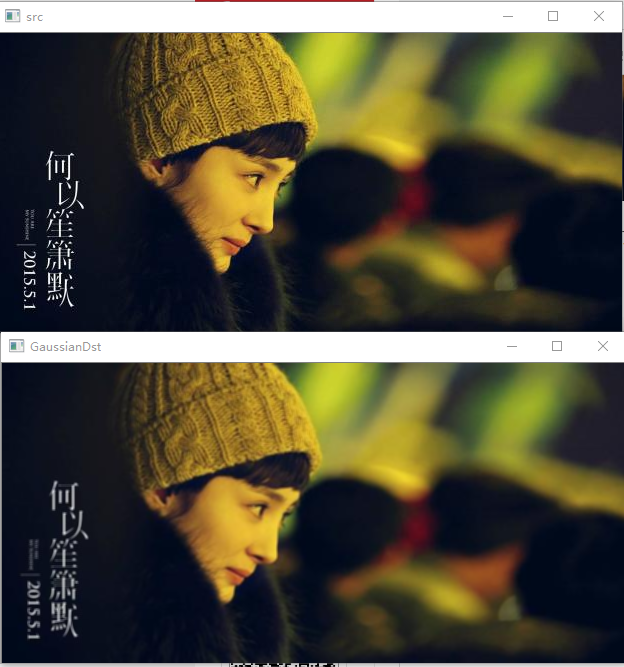3、椒盐滤波。

Mat src=imread("D:\\test2.png");
if(src.empty())
{
return;
}
Mat blurDst;
medianBlur(src,blurDst,3);
imshow("medianDst",blurDst);
imshow("src",src);
waitKey(-1);

结果如下：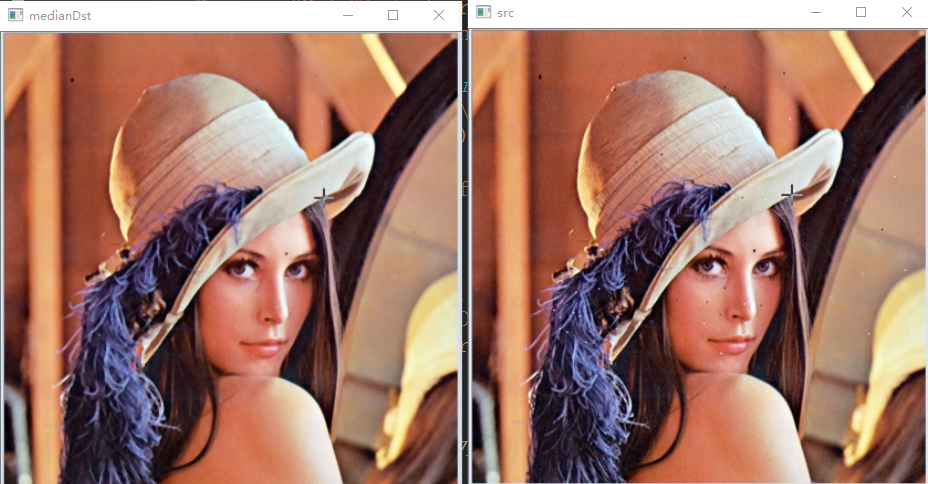我们可以看到，去掉了一些噪声，当然我们还可以调一下size的大小，看看效果。

4、双边滤波。

   Mat src=imread("D:\\test2.png");
if(src.empty())
{
return;
}
Mat blurDst;
bilateralFilter(src,blurDst,15,100,3);
imshow("bilateralDst",blurDst);
imshow("src",src);
waitKey(-1);

结果如下：总结：

均值滤波无法克服边缘像素信息丢失的缺陷，原因是均值滤波是基于平均权重。

高斯滤波虽然克服了均值滤波的缺陷，但是无法完全避免，因为没有考虑像素值得不同，只是基于空间分布考虑。

双边滤波避免了边缘信息丢失，保留了图像的轮廓不变。


展开全文• 通常，图像处理软件会提供"模糊"（blur）滤镜，使图片产生模糊的效果。   "模糊"的算法有很多种，其中有一种叫做"高斯模糊"（Gaussian Blur）。它将正态分布（又名"高斯...高斯模糊 图像处理
• 只对高斯与散焦模糊的非盲去卷积领域，对于运动模糊并未做总结，但实际上除了点扩散函数的估计有区别，实际上这三类去模糊甚至和去噪，损失函数模型都可以通用。 这里对于只做一家之言，如有反对意见请留言指正： ...
• 我们前面提到，使用平均操作或加权平均操作可以降低图像的噪声，并由此引出了空间...下面是一张哈勃望远镜拍摄的星空原始图像(来自冈萨雷斯的《数字图像处理)，我们将这张图像和使用平滑滤波器后生成的图像进行一下图像处理 opencv
• 在数字图像上进行平滑操作，就是需要用模板(高斯模板，均值模板)在图像上做卷积，得到新值，这就是模糊的原理。卷积核中的每个元素叫做卷积系数，卷积核中卷积系数的大小、方向及排列次序决定图像卷积后处理的最终...高斯模糊
• 标志绿黄红是颜色感觉的含混描述，要用模糊形式表达，可通过隶属度定义颜色（光的波长）的函数实现。输入的隶属度函数如下图： 上面提到的颜色和成熟度关系的知识可用下面的IF-THEN模糊规则给出： IF-THEN规则...图像处理
• Smooth/Blur（平滑和模糊） 是图像处理中最简单和常用的操作之一 使用该操作的原因之一就是为了给图像预处理时候降低噪声 ， 把噪声与周围的数值平均一下就可以平滑噪声。 使用Smooth/Blur操作背后是数学的卷积计算 ...
• 视频图像模糊常用处理方法 随着“平安城市”的广泛建设，各大城市已经建有大量的视频监控系统，虽然监控系统己经广泛地存在于银行、商场、车站和交通路口等公共场所，但是在公安工作中，由于设备或者其他条件的...
• 本章主要讲图像处理中的模糊处理部分 英文叫做blur, 也叫做smootiing, 中文中叫做模糊或者平滑。 用过photoshop的人都应该知道，滤镜里面就有模糊这个选项，我们现在看看它是怎么实现的。 一含义  模糊...
• Python+OpenCV3.3图像处理视频培训课程：该教程基于Python3.6+OpenCV新版本3.3.0详细讲述Python OpenCV图像处理部分内容，包括opencv人脸识别、人脸检测、数字验证码识别等内容。是Python开发者学习图像知识与应用...
• 运动模糊产生：假设图像f(x,y)进行平面运动，x0(t)和y0(t)分别是在x和y方向上随时间变化的量。则有表达式如下，g(x,y)为转换后该点的灰度值，f(x,y)为原图中对应点的灰度值。 计算后的g(x,y)值将大于255，因此这里...数字图像 运动模糊 opencv
• 最近在做人脸识别清洗样本的工作，发现经过人脸对齐后存在部分图片...图片越模糊，imageVar的值越小，图像模糊。 #-*-coding:utf-8-*- import sys #reload(sys) #sys.setdefaultencoding('utf-8') import os i...
• 本文选自：http://www.cnblogs.com/Imageshop/p/3302850.html，作者写的非常不错，包括另外一篇文章：http://www.cnblogs.com/Imageshop/p/3307308.html，感谢作者的辛勤劳动！自己整理过来备份。...
...